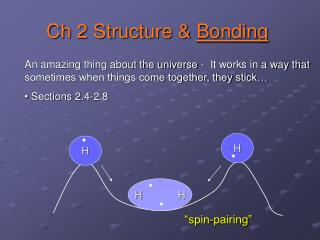DownloadDownload PresentationH H

# H H

Download Presentation## H H

- - - - - - - - - - - - - - - - - - - - - - - - - - - E N D - - - - - - - - - - - - - - - - - - - - - - - - - - -
##### Presentation Transcript

1. H H H H Ch 2 Structure & Bonding • An amazing thing about the universe - It works in a way that sometimes when things come together, they stick… • Sections 2.4-2.8 “spin-pairing”

2. Valence Bonding (Localized) vs. Molecular Orbital Theory (Delocalized)

3. Orbital Overlap (Localized Bonding) • Bonding orbitals are constructed by combining atomic orbitals from adjacent atoms. • In VB Theory, usually only worry about the valence electrons • From Quantum Mechanics: orbitals can add or subtract; therefore constructive or destructive interference is possible

4. Localized bonding… H2 Orbital Overlap: As two H atoms approach, the overlap of their 1s atomic orbitals increases. The wave amplitudes add, generating a new orbital with high electron density between the nuclei.

5. Sigma bonding: The s bond is actually symmetry notation • means bonding is directed along internuclear axis • sigma bonds have a C∞ axis

6. Pi bonding: The p bond is also found in symmetry notation • means bonding is above & below the internuclear axis

7. PH3 Phosphine is a colorless, highly toxic gas with bond angles of 93.6°. Describe the bonding in PH3.

8. Oh shoot… What about methane? What is the electron configuration of methane?

9. s and p hybridization Promotion: excitation of an electron to a higher energy orbital in the course of bond formation – not real exactly Hybridization: mathematical mixing (linear combinations) of valence atomic orbitals to achieve new equal energy degenerate orbitals

10. Methane hybridization

11. CH4 is tetrahedral • Therefore, 2s and the 2px, 2py, & 2pz must hybridize • new orbitals are called sp3 • An inner atom with a steric number of 4 has tetrahedral electron group geometry and can be described using sp3 hybrid orbitals.

12. General Features of Hybridization • The # of valence orbitals generated by hybridization equals the # of valence AOs participating in hybridization. • The steric number of an inner atom uniquely determines the number and type of hybrid orbitals. • Hybrid orbitals form localized bonds by overlap with atomic orbitals or with other hybrid orbitals. • There is no need to hybridize orbitals on outer atoms, because atoms do not have limiting geometries. The bonds formed by all other outer atoms can be described using valence p orbitals.

13. Isolobal: When analogous fragments on differing molecules have closely similar bonding patterns

14. Molecular Orbital Theory: When overlapping AOs just won’t cut it. • So far, bonding has been described as overlapping AOs or hybrid orbitals • However, *all* electrons from each bonding atom feel the presence of the others • Bonding in the diatomic molecules second row elements can be explained in two ways. • Localized Bonding (LB) Theory, a.k.a. Valence Bond Theory • Molecular Orbital (MO) Theory • MO Theory • assumes pure s and p AOs of the atoms in a molecule combine to produce orbitals that are spread out, or delocalized, over several atoms, leading to MOs • One advantage over VB Theory: correctly explains electronic structures of molecules which do not follow Lewis Dot structure.

15. 4 Principles of MO Theory 1st Principle: the total # of MOs produced by a set of interacting AOs is equal to the # of interacting orbitals 2nd Principle: the bonding MO is lower in energy than the parent AOs & the antibonding MO is higher in energy (LCAO) To explain this, let’s look at the hydrogen molecule, H2

16. Bonding and Antibonding in H2 • Each hydrogen atom contributes a 1s orbital • These orbitals can be added or subtracted • Addition: Bonding MO (s1s) • Subtraction: Bonding MO (s1s*)

17. Principles of MO Theory 3rd Principle: electrons of the molecule are assigned to orbitals of successively higher energy according to the Aufbau principle and Hund’s Rule • Electrons occupy the lowest energy orbitals first. • Atoms are most stable with the highest number of unpaired electrons

18. molecular orbital diagram of He2 NOW: Use a molecular orbital diagram to predict if it is possible to form the He2+ cation.

19. Bond Order in MO Theory • Bond order allows us to represent the net amount of bonding between two atoms. • The higher the bond order, the more stable the structure

20. One More Principle… 4th Principle: atomic orbitals combine to form molecular orbitals most effectively when the atomic orbitals are of similar energy & symmetry • i.e. a 1s will not bond with a 2s.

21. Second-Row Diatomic Molecules - subtractive additive +

22. NOTE NUMBERING!

23. Orbital Mixing • In B2, the overlap of 2s and 2pz orbitals stabilizes ss and destabilizes sp • The amount of mixing depends on the energy difference between the 2s and 2p atomic orbitals. • Mixing is largest when the energies of the orbitals are nearly the same 2 cases: 1) Zave ≤ 7, 2) Zave > 7 (qualitative)

24. Heteronuclear Diatomic Molecules Let’s examine the MO diagram of NO …and of CO…

25. Evidence for Antibonding Orbitals